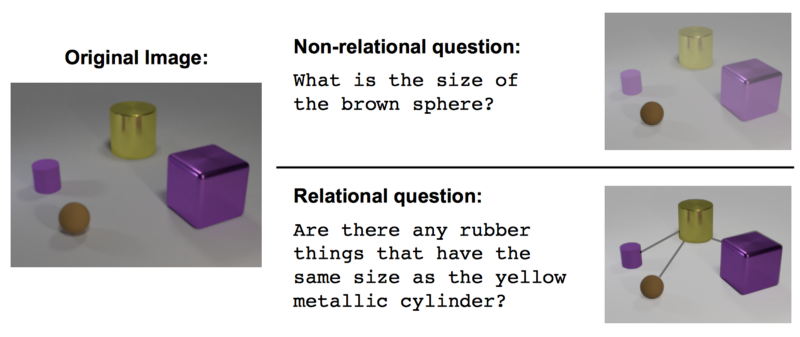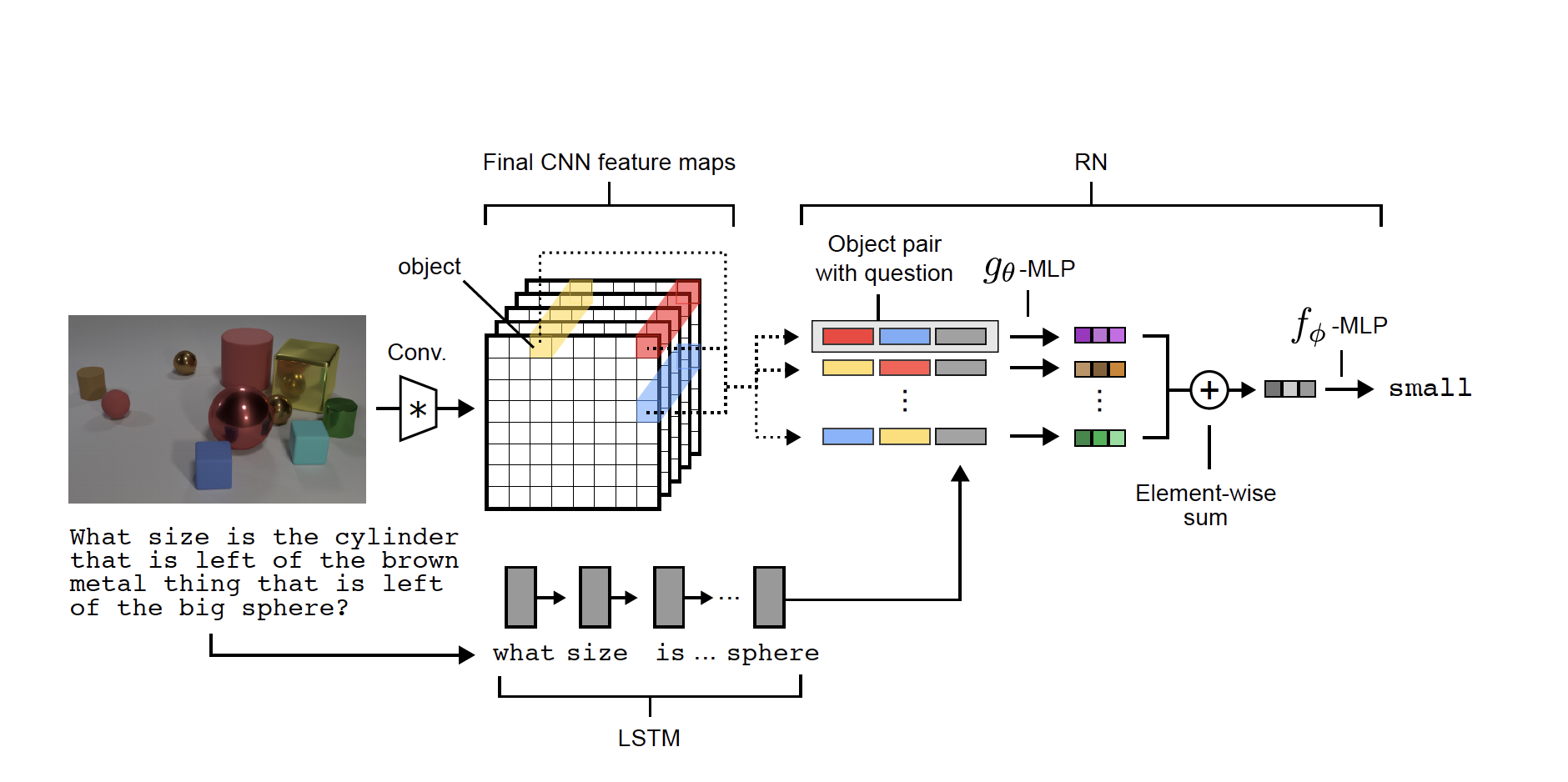## Relational Network

Overview of Adam Santoro et al. (NeurIPS 2017).

Considering that most of the data is some form of graph, there has been lot of focus on improving neural networks to work with graph data. Amidst this, Santoro et al, paper focuses on neural network’s ability of doing relational-reasoning i.e. manipulating structured representations of entities and relations. What separates this paper from the other graph network papers is two things: a) the graph or relation between entities is not provided rather learned and b) the edges between entities can be of different types. Most graph network papers that learn edges focus on approximating a distance metric between entities. This paper instead focuses on learning relations between entities.

### Summary

The paper proposes a computational block which they call relational network (RN), which takes a set of object ($O$) as input and outputs a vector. The main computational unit in RN are functions: $f_\phi$ and $g_\theta$, which are both Multi Layered Perceptrons. $g_\theta$ approximates the relation between each pair of object and $f_\phi$ performs the reasoning over these entities.

$$\operatorname{RN}(O)=f_{\phi}\left(\sum_{i, j} g_{\theta}\left(o_{i}, o_{j}\right)\right)$$

To achieve combinatorial generalization, i.e. be able to use $f_\phi$ over varying number of objects, authors use the sum of $g_\theta$ as input to $f_\phi$. So, the the input dimension of $f_\phi$ is equal to the output dimension of the $g_\theta$, which are both constant and independent of order or number of objects in the input.

RN can also be thought of as a MLP with parameter typing for first few hidden layers, which is equivalent to $g_\theta$.

Making the input and output dimensions independent of the number of objects has a big advantage in terms of data efficiency. In standard MLP, when the number of objects increase, the input dimension may increase and hence the number of parameters would also increase.

The whole RN network is end-to-end differentiable and hence trainable by back propagation.

### RN for VQA

Authors show the utility of RN on Visual Question Answering task of CLEVR dataset. In CLEVR, a model needs to reason about relations between different objects in the image and then answer the question.In the figure above, even though the question ‘‘What is the size of the brown sphere?’’ is shown as non-relational, if the answer of this question is going to be ‘small’, ‘medium’ or ’large’, I would consider it as a relational question. Because the size is relative. On other hand, if the answer is ‘2 cm in diameter’ it is non-relational. I strongly believe the dataset is aiming for the former answer.

Input image is processed through a CNN to obtain object embedding. Input questions are processed though LSTM to obtain question embedding. The proposed $g_\theta$ function is then modified to predict the relationship between objects in context of the question asked: $g_\theta(o_i, o_j, q)$. $Here, q_\theta$ generates a fixed length vector which are aggregated and forwarded to $f_\phi$ which outputs softmax over all the possible answers.RN’s success in Sort-of-CLEVR dataset show that it is able to do better relational reasoning then MLP.

### Critique

In my opinion since the embedding of the relation i.e. output of $g_\theta$ are not evaluated here, the claim of RN being able to do relational reasoning is not accurate. Experiments clearly show the benefit of the CNN+RN over CNN+MLP but that just means RN is better at fitting the curve.

I do not quite understand what entails relational reasoning and how can one purely test that ability.

1. Adam Santoro, David Raposo, David G.T. Barrett, Mateusz Malinowski, Razvan Pascanu, Peter Battaglia, and Timothy Lillicrap, A simple neural network module for relational reasoning, NeurIPS 2017
2. https://rasmusbergpalm.github.io/recurrent-relational-networks/